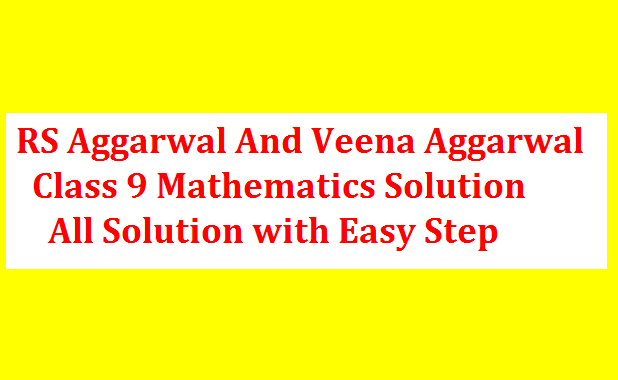## RS Aggarwal And Veena Aggarwal Class 9 Math Sixth Chapter Introduction to Euclid’s Geometry Exercise 6 SolutionRS Aggarwal And Veena Aggarwal Class 9 Math Sixth Chapter Introduction to Euclid’s Geometry Exercise 6 Solution EXERCISE 4B (1) (i) An axiom is true statement used throughout mathematics and not specifically linked to geometry. A statement that requires a proof is called a theorem. (ii) Theorems are naturally challenged more than axioms. (iii) Theorems […]